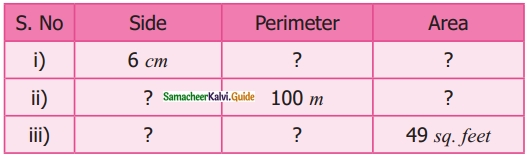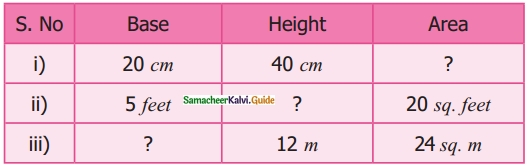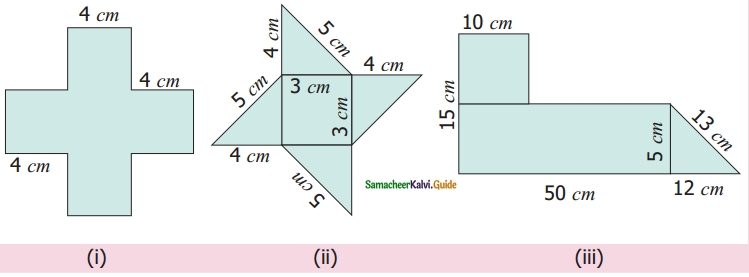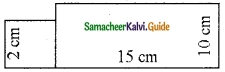Students can download Maths Chapter 3 Perimeter and Area Ex 3.1 Questions and Answers, Notes, Samacheer Kalvi 6th Maths Guide Pdf helps you to revise the complete Tamilnadu State Board New Syllabus, helps students complete homework assignments and to score high marks in board exams.

## Tamilnadu Samacheer Kalvi 6th Maths Solutions Term 3 Chapter 3 Perimeter and Area Ex 3.1

Question 1.
The table given below contains some measures of the rectangle. Find the unknown values.Solution:

(i) Area of the rectangle = (length × breadth) sq unit.
Perimeter of a rectangle = 2(1 + b) units.
l = 5 cm
b = 8 cm
∴ p = 2 (l + b) cm = 2 (5 + 8) cm = 2 × 13 cm
p = 26 cm
Area = (l × b) cm2 = (5 × 8) cm2
A = 40 cm2

(ii) l = 13 cm
p = 54 cm
Perimeter = 2 (l + b) units
54 = 2 (13 + b) cm
$$\frac{54}{2}$$ = 13 + b
27 = 13 + b
b = 27 – 13
b = 14 cm
Area = l × b sq. unit = 13 × 14 cm2
A = 182 cm2

(iii) b = 15 cm
p = 60 cm
p = 2 (l + b) units
60 = 2 (l + 15) cm
$$\frac{60}{2}$$ = l + 15
30 = l + 15
l = 30 – 15 .
l = 15 cm
Area = l × b unit2 = 15 × 15 cm2 = 225 cm2
A = 225 cm2

(iv) l = 10 m
Area = 120 sq metre
Area = l × b sq.m
120 = 10 × 6
b = $$\frac{120}{10}$$
b = 12 m
Perimeter =2 (l + b) units = 2(10 + 12) units = 2 × 22 m
A = 44 m

(v) b = 4 feet.
Area = 20 sq. feet
Area = l × b sq .feet
20 = l × 4
l = $$\frac{20}{4}$$ feet
l = 5 feet
Perimeter = 2 (l + b) units.
p = 2 (5 + 4) feet = 2 × 9
p = 18 feet

Question 2.
The table given below contains some measures of the square. Find the unknown values.Solution:
(i) 24 cm, 36 cm²
(ii) 25 m, 625 m²
(iii) 7 feet, 28 feetQuestion 3.
The table given below contains some measures of the right angled triangle. Find the unknown values.Solution:

Area of the right triangle = $$\frac{1}{2}$$ × (base × height) unit2
(i) b = 20 cm
h = 40 cm
Area = $$\frac{1}{2}$$ (b × h) cm2 = $$\frac{1}{2}$$ × 20 × 40 = 400 cm2
A = 400 cm2

(ii) b = 5 feet
Area = $$\frac{1}{2}$$ × b × h unit2
= 20 = $$\frac{1}{2}$$ × 5 × h sq. feet
$$\frac{20 \times 2}{5}$$ = h
h = 8 feet

(iii) Area = $$\frac{1}{2}$$ × (base × height) unit2
24 = $$\frac{1}{2}$$ × b × 12 m2
base = $$\frac{24 \times 2}{12}$$ m = 4 m
Base = 4m

Question 4.
The table given below contains some measures of the triangle. Find the unknown values.Solution:
(i) 13 cm
(ii) 6 m
(iii) 8 feet

Question 5.
Fill in the blanks.
(i) 5 cm² = ______ mm²
(ii) 26 m² = ______ cm²
(iii) 8 km² = ______ m²
Solution:
(i) 500
(ii) 260000
(iii) 8000000Question 6.
Find the perimeter and area of the following shapes.Solution:
(i) Perimeter = (4 + 4 + 4 + 4 + 4 + 4 + 4 + 4 + 4 + 4 + 4 + 4)
= 48 cm
a = 4 cm
Area of 1 square = 4 × 4 cm²
= 16 cm²
Area of 5 squares = 5 × 16 cm²
= 80 cm²

(ii) Perimeter = (4 + 5 + 4 + 5 + 4 + 5 + 4 + 5)
= 36 cm
Area of 1 triangle = $$\frac{1}{2}$$ × b × h sq units
= $$\frac{1}{2}$$ × 4 × 5 cm²
= 10 cm²
Area of 4 triangles= 4 × 10 cm²
= 40 cm²
Area of the square = 3 × 3 cm²
= 9 cm²
Total area = (40 + 9) cm²
= 49 cm²(iii) Perimeter = (15 + 50 + 12 + 13 + 10 + 10 + 40)
= 150 cm
Area of the square = 10 × 10 cm²
= 100 cm²
= 250 cm²
Area of the triangle = $$\frac{1}{2}$$ × 12 × 5 cm²
= $$\frac{1}{2}$$ × 126 x 5 cm²
= 30 cm²
Total area = (100 + 250 + 30) cm²
= 380 cm²

Question 7.
Find the perimeter and the area of the rectangle whose length is 6 m and breadth is 4m?
Solution:
l = 6 m, b = 4 m Perimeter of the rectangle
= 2 (l + b) units
= 2 (6 + 4) m
= 2 (10) m
= 20 m
Area of the rectangle = l × b sq units
= 4 × 6 m²
= 24 m²Question 8.
Find the perimeter and area of a square whose side is 8 cm.
Solution:
a = 8 cm
The perimeter of a square
= 4a units
= 4 × 8 cm
= 32 cm
Area of the square = a × a sq units
= 8 × 8 cm²
= 64 cm²

Question 9.
Find the perimeter and the area of right angled triangle whose sides are 6 feet, 8 feet and 10 feet.
Solution:
Perimeter of the triangle
= (a + b + c) units
= (6 + 8 + 10) feet
= 24 feet
Area of the triangle = $$\frac{1}{2}$$ × b × h sq units
$$\frac{1}{2}$$ × 6³× 8 feet square = 24 sq. feetQuestion 10.
Find the perimeter of
(i) A scalene triangle with sides 7 m, 8 m, 10 m.
(ii) An isosceles triangle with equal sides 10 cm each and third side is 7 cm.
(iii) An equilateral triangle with a side of 6 cm.
Solution:
(i) Perimeter of the triangle
= (a + b + c) units
= (7 + 8 + 10) m
= 25

(ii) Perimeter of the triangle
= (10 + 10 + 7) cm
= 27 cm

(iii) Perimeter of the triangle
= (6 + 6 + 6) cm
= 18 cmQuestion 11.
The area of a rectangular shaped photo is 820 sq. cm. and its width is 20 cm. What is its length? Also find its perimeter.
Solution:
Given Area = 820 cm²
Width = 20 cm
Area of the rectangle
= l × b sq. units
820 = l × 20
$$\frac{820}{20}$$ = l
41 = l
length l = 41 cm
Perimeter = 2(l + b) units
= 2(41 + 20) cm
= 2(61) cm
= 122 cm

Question 12.
A square park has 40 m as its perimeter. What is the length of its side? Also find its area.
Solution:
perimeter = 40 m
4a = 40 m
a = $$\frac{40}{4}$$
Side a = 10 m
Area = a × a sq units
= 10 × 10 m²
= 100 m²Question 13.
The scalene triangle has 40 cm as its perimeter and whose two sides are 13 cm and 15 cm, find the third side.
Solution:
Let the third side be C
perimeter = (a + b + c) units
40 = 13 + 15 + C
40 = 28 + C
C = 40 – 28
C = 12 units
C = 12 cm

Question 14.
A field is in the shape of a right angled triangle whose base is 25 m and height 20 m. Find the cost of levelling the field at the rate of Rs 45/- per sq.m²
Solution:
b = 25 m, h = 20 m
Area of the triangle = $$\frac{1}{2}$$ × bh sq.units
= $$\frac{1}{2}$$ × 25 × 20 m²
= 250 m²
Cost of levelling 1 m² = Rs 45
Cost of levelling 250 m² = Rs 45 × 250
= Rs. 11250

Question 15.
A square of side 2 cm is joined with a rectangle of length 15 cm and breadth 10 cm. Find the perimeter of the combined shape.
Solution:Perimeter of the combined shape = Lengths of the outer boundaries
= (15 + 10 + 2 + 2 + 2 + 13 + 10) cm = 54 cm
Perimeter = 54 cmObjective Type Questions

Question 16.
The following figures are of equal area. Which figure has the least perimeter?Solution:
(b)

Question 17.
If two identical rectangles of perimeter 30 cm are joined together, then the perimeter of the new shape will be
(a) equal to 60 cm
(b) less than 60 cm
(c) greater than 60 cm
(d) equal to 45 cm
Solution:
(b) less than 60 cmQuestion 18.
If every side of a rectangle is doubled, then its area becomes _____ times
(a) 2
(b) 3
(c) 4
(d) 6
Solution:
(c) 4
2l × 2b = 4l × b

Question 19.
The side of the square is 10 cm. If its side is tripled, then by how many times will its perimeter increase?
(a) 2 times
(b) 4 times
(c) 6 times
(d) 3 times
Solution:
(d) 3 timesQuestion 20.
The length and breadth of a rectangular sheet of paper are 15 cm and 12 cm respectively. A rectangular piece is cut from one of its corners. Which of the following statement is correct for the remaining sheet?
(a) Perimeter remains the same but the area changes
(b) Area remains the same but the perimeter changes
(c) There will be a change in both area and perimeter
(d) Both the area and perimeter remains the same.
Solution:
(a) Perimeter remains the same but the area changes